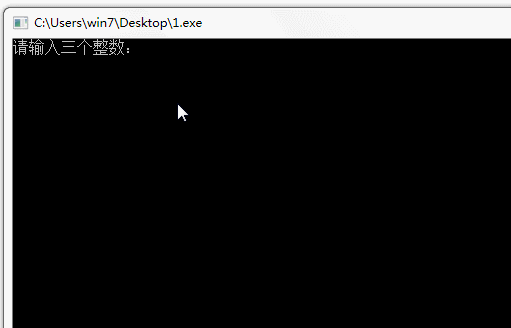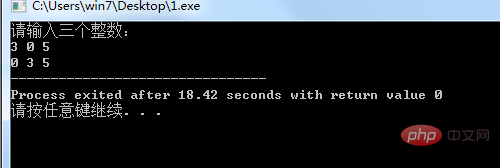# c语言怎么输入3个数并从小到大输出php入门到就业线上直播课：进入学习

scanf 是 scan format 的缩写，意思是格式化扫描，也就是从键盘获得用户输入

`scanf("%d%d%d",&a,&b,&c);`

```	if(a>b){
t=a;
a=b;
b=t;
}
if(a>c){
t=a;
a=c;
c=t;
}
if(b>c){
t=b;
b=c;
c=t;
}```

printf() 是 C 语言标准库函数，用于将格式化后的字符串输出到标准输出。标准输出，即标准输出文件，对应终端的屏幕。printf() 申明于头文件 stdio.h。

`printf("%d %d %d",a,b,c);`

```#include <stdio.h>
int main(){
int a,b,c,t;
printf("请输入三个整数：\n");
scanf("%d%d%d",&a,&b,&c);
if(a>b){	// 3 2 1
t=a;	// t=3
a=b;	// a=2
b=t;	// b=3
}
if(a>c){
t=a;	// t=2
a=c;	// a=1
c=t;	// c=2
}
if(b>c){
t=b;	// t=3
b=c;	// b=2
c=t;	// c=3
}
printf("%d %d %d",a,b,c);
return 0;
}```1/1